# Power BI: Is Leap Year Pattern

### Colin Maitland, 16 August 2021

In this article, I will demonstrate several DAX formulas for Power BI that may be used to calculate whether a date falls within a Leap Year.

Here is a table of dates showing the results for a set of dates. You will notice that:

· If the Date is blank, then the result is False.

· If the Date falls within a century year that is evenly divisible by 400, such as the year 2000, then the result is True.

· If the Date falls within a century year that is not evenly divisible by 400, such as the year 2100, then the result is False.

· Otherwise, if the Date falls within a year that is evenly divisible by 4 then the result is True, otherwise the result is false.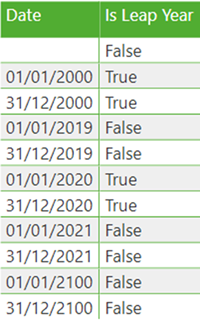Here are two DAX formulas that may be used to calculate this result. When using these formulas, you simply replace the reference to Value with a reference to a Column, Function or Measure such as ‘Date’[Date], TODAY(), or [Date] respectively. You can then return the result or use the result in a subsequent part of the formula.

Short Formula

This is an example of a short formula that calculates whether the date falls within a Leap Year. This formula checks if the date is not blank and the difference in days between 28th February and 1st of March in the year in which the date falls is 2 days. If so, then the date falls within a Leap Year, otherwise it does not. This formula also works without the outer ‘IF ( NOT ISBLANK ( _DATE ), …, FALSE() )’ clause because the Year function returns 1900 for a blank date.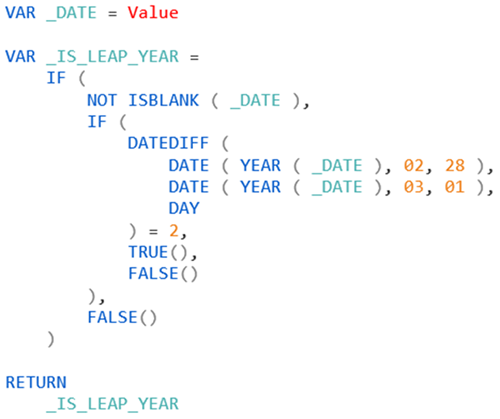Long Formula

This is an example of a long formula that calculates whether the date falls within a Leap Year. This formula checks if the date is not blank, is evenly divisible by 4 and is not evenly divisible by 400. If so, then the date falls within a Leap Year, otherwise it does not. This formula does not work without the outer ‘IF ( NOT ISBLANK ( _DATE ), …, FALSE() )’ clause.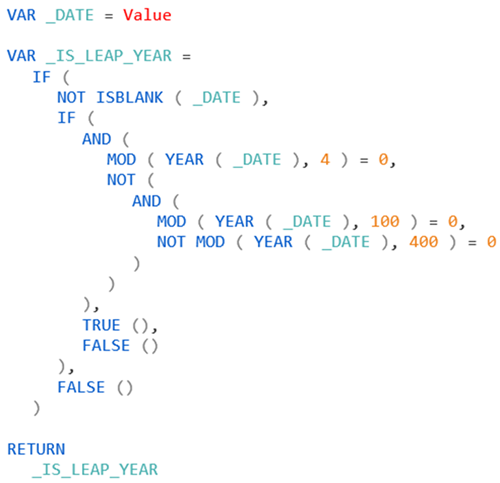Alternate Formulas

Both of these formulas can be altered so that a blank instead of False is returned for a blank date. It is not possible to do this using the previous formulas because the result returned by those formulas are always a True/False data type. Because of this, attempting to return Blank() will always be returned as False.

However, the formulas below will return these results.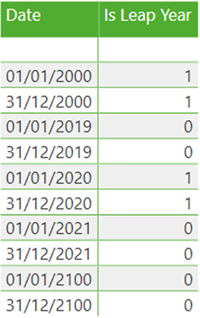Alternate Short Formula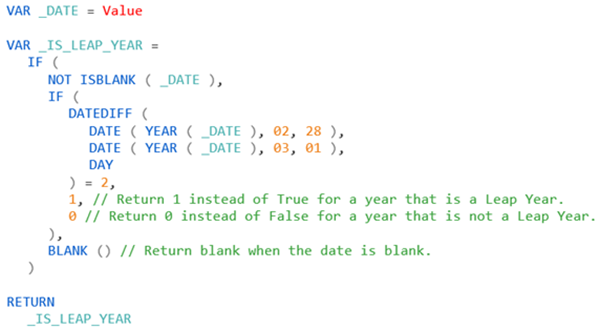Alternate Long Formula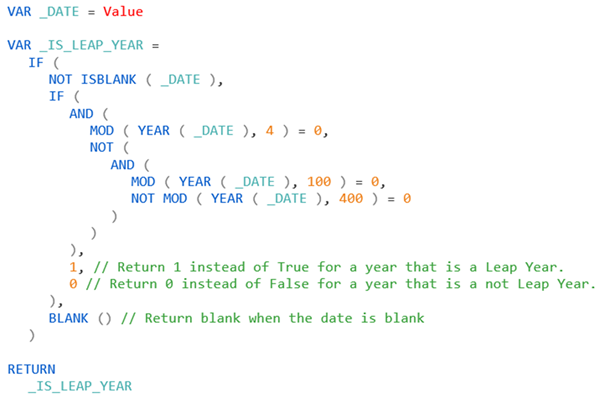Example – Moving Annual Total (MAT)

In many instances, the use of an Is Leap Year calculation is part of a larger formula. Here is an example of a formula for a Calculation Item that calculates a Moving Annual Total (MAT) for the selected Measure.

This formula calculates its result in such a way that the result always includes the 365 days on or before the highest selected date plus the 29th February if the year of the highest selected date is a Leap Year and the highest selected date is >= 29th February or the previous year to the highest selected date is a Leap Year and the highest selected date is <= 28th February.

Can you identify which of the two previous formulas has been used? Can you identify how many times the formula is used?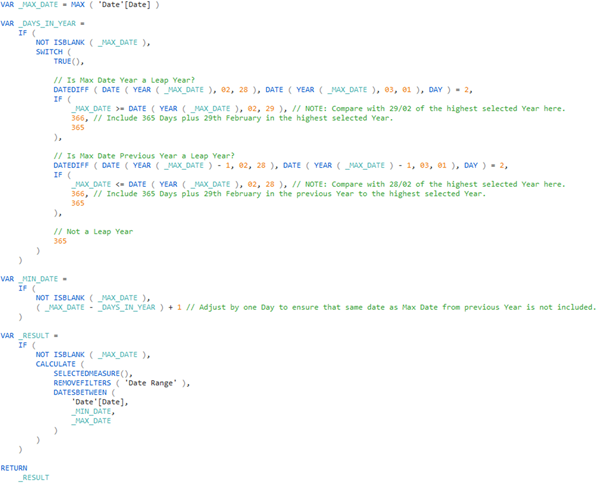In this example, if there was no need to ensure that 365 days plus the 29th of February needed to be included in this calculation, then the VAR _DAYS_IN_YEAR = part of the formula could simply be changed to VAR _DAYS_IN_YEAR = 365.

Conclusion

In conclusion, the calculation of whether a date falls within a Leap Year in a DAX formula is a common pattern. In this article, I have demonstrated four possible patterns and the use of one of these patterns within a larger encapsulating DAX formula. The first two patterns return True for dates that fall within a Leap Years, False for dates that do not fall within a Leap Years and False for blank dates. The alternate patterns return 1 for dates that fall within a Leap Year, False for dates that do not fall within a Leap Year and blank for blank dates.